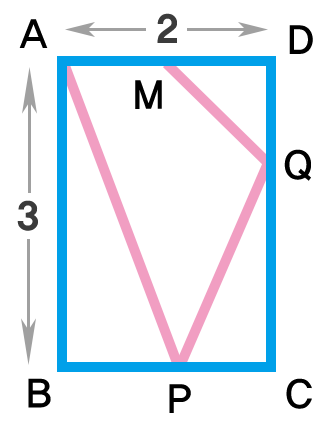# Shortest Path #2

Geometry Level 3There is a rectangle $ABCD$ with side lengths 2 and 3 as shown on the right.

Point $P$ is on $\overline{BC}$ and point $Q$ is on $\overline{CD}.$

$M$ is the midpoint of $\overline{AD}.$

Let $m$ be the length of the shortest path from $A$ to $M,$ while visiting $P$ and $Q$ sequentially.

Find the value of $m^2.$

This problem is a part of <Shortest Path> series.

×